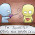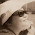## Wednesday, 6 March 2013

### Let's try to explain these using 'rectangles'

1. Choose ONE question from EACH group
2. Use the "rectangle method" to explain how to simplify each expression.
3. Also show how you would use distributive law to simplify each expression.

• Complete both questions in the same sheet of paper.
• Write down the question number clearly.
• Label the diagrams neatly. It should have its 'dimensions/values' clearly indicated at each 'part' of the rectangles.
Submission
Take a picture of your work and post it in this Maths blog by today 2359h.

• Subject title: Expansion (Q1a & Q2c) by ....
• Label: Algebra, Expansion

Group 1 Questions
Q1a. (a + 3)(a + 5)
Q1b. (3m + n)(2m + 5n)
Q1c. (5x + 3)^2
Q1d. (a^2 + a + 2)(a + 3)

Group 2 Questions
Q2a. (b + 4)(b - 1)
Q2b. (c + 4d)(c - 2)
Q2c. (b + 4)(h - k)
Q2d. (2a + 3b) (a - 4c)

1.why only group 1 and 2?

1.Miss Loh wrote choose 1 question from each grp. But we are not going according to any grp so we can choose which questions we want to do.

2.@Victoria

Yes, you are right in interpreting the question :)